# Examples for Bayesian Mediation Analysis

## Package installation

The R package BayesianMediationA is created for linear or nonlinear mediation analysis with binary, continuous, or time-to-event outcomes under the Bayesian setting Yu and Li (2022). The vignette is composed of three parts. Part I focuses on the data sets used for examples, and part II on how to transform variables and prepare data for the mediation analysis. Part III walks through the function on Bayesian mediation analysis, and explains how to make inferences on mediation effects of interests.

To use the R package BayesianMediationA, we first install the package in R (install.packages("BayesianMediationA")) and load it.

## The Data Set

We use the data set weight_behavior’ which is included in the package as examples for mediation analysis with binary or continuous outcomes . In addition, a dataset is generated for the time-to-event outcome as the following:

#use a simulation
set.seed(1)
N=100

alpha=0.5
x=rnorm(N,0,1)
x=ifelse(x>0,1,0) #the binary exposure. If want to use a continuous exposure, remove this line
e1=rnorm(N,0,1)
M=alpha*x+e1   #the mediator
lambda=0.01
rho=1
beta=1.2
c=-1
rateC=0.001
v=runif(n=N)
Tlat =(- log(v) / (lambda * exp(c*x+M*beta)))^(1 / rho) #the event time
C=rexp(n=N, rate=rateC)  #the censoring time
time=pmin(Tlat, C)
status <- as.numeric(Tlat <= C)
example2 <-cbind(x, M, time, status) #the dataset

## Data Transformation and Organization

The $$data_org$$ function is used to do the transformation before the mediation analysis. In the function, the exposure variable(s) ($$pred$$) and the mediator(s) ($$m$$) are required to input. The response variable ($$y$$) is also required. If $$y$$ is binary or categorical, its reference level is input in the argument $$levely$$. Similarly, the reference levels for the exposure variables and mediators are input in the argument $$predref$$ and $$mref$$ respectively.

Other input data include $$cova$$, the covaritates that are used to explain $$y$$, and $$mcov$$, the covariates for mediators. Covariates for $$y$$ are defined as predictors for $$y$$, but not explained by the exposure variables $$pred$$. Covariates for mediators are explanatory variables for mediators other than the exposure variable(s). Accompanying $$mcov$$, we have $$mclist$$ to specify different covariates for different mediators. If $$mclist$$ is NULL (by default), all covariates in $$mcov$$ are used for all mediators in $$m$$. Otherwise, the first item of $$mclist$$ lists all column numbers/names of mediators in $$m$$ that are to be explained by covariates in $$mcov$$, the following items give the covariates in $$mcov$$ for the mediators in the order of the first item. $$NA$$ is used when no covariance is to be used for the corresponding mediator. For example, mclist=list(c(2,4),NA,2:3)’ means that all mediators use all covariates in $$mcov$$, except for the second mediator which use none of the covariates, and the fourth mediator, which uses only columns 2 to 3 of $$mcov$$ as its covariates.

Variables can be transformed to denote potential nonlinear relationships. The transformation functions are expressed in arguments $$fpy$$, $$fmy$$, and $$fpm$$ separately. In the name of the arguments, the p' stands for the predictors,m’ mediators, and y’ the outcome. Namely, $$fpy$$ define the transformation functions of the predictors in explaining the outcome $$y$$. The first item lists column numbers/variable names of the exposure variable in $$pred$$, which needs to be transformed to explain $$y$$. By the order of the first item, each of the rest items of $$fpy$$ lists the transformation functional expressions for the predictor. The exposures/predictors not specified in the list will not be transformed in any way in explaining y. For example, list(1,c(“x^2”,“log(x)”)) means that the first column of the pred will be transformed to square and log forms to explain $$y$$. The $$fmy$$ is defined the same way for the transformed mediators to explain the outcome variable $$y$$.

$$fpm$$ denotes the transformation-function-expression list on exposure variable(s) ($$pred$$) in explaining mediators ($$m$$). The definition of $$fpm$$ is similar to those of $$fpy$$ and $$fmy$$ except that the first item is a matrix with two columns: the first column is the column numbers of the mediators in $$m$$, which should be explained by the transformed predictor(s) indicated by the second column. The second column indicates the column number of the exposure in $$pred$$ that will be transformed to explain the mediator identified by the 1st column of the same row. By the order of the rows of the first item, each of the rest items of fpm lists the transformation functional expressions for the exposure (identified by column 2) in explaining each mediator (identified by column 1). The mediators not specified in the list will be explained by the original format of the exposures in $$pred$$. For example, fpm=list(matrix(c(1,2,1,1),2,2), “x2”,c(”x”,”x2”))’ means that $$pred[,1]^2$$ is used to explain $$m[,1]$$, and both $$pred[,1]$$ and $$pred[,1]^2$$ are used to explain $$m[,2]$$.

Finally, deltap and deltam define the change in predictors or mediators respectively in calculating the mediation effect.Yu et al. (2022)

Users do not run the $$data\_org$$ function by itself. All arguments are included in the main Bayesian mediation analysis function $$bma.bx.cy$$, which runs the $$data\_org$$ function in it to organize data for mediation analysis.

## The function $$bma.bx.cy$$ for Bayesian mediation analysis

The function $$bma.bx.cy$$ are used to perform the Bayesian mediation analysis. In the function, the $$data\_org$$ function is called first, which involves all arguments described above. In addition, prior distributions in the generalized linear models can be set up. By default, all coefficients in the Bayesian generalized linear models are independently normal distributed with mean $$0$$ and the precision term specified by $$speci$$, default at $$10^{-6}$$.

The prior means and the variance-covariance matrix can be altered. $$mu$$ defines the prior mean for coefficients of mediators, $$muc$$ the prior mean vector (of length $$p2$$) for coefficients of exposure(s), and $$mucv$$ the prior mean vector for coefficients of covariate(s)in the final model for $$y$$. Related, $$Omega$$, $$Omegac$$, and $$Omegacv$$ defines the prior variance-covariance matrix for the coefficients of mediators, exposure(s), and covariates respectively.

The prior distributions for coefficients of intercept/covariates, and exposures in explaining mediators are also assumed to be normal. The default prior mean and variance-covariance matrix are as above, and can be changed in $$mu0.1$$ and $$mu1.1$$ for means, and $$Omega0.1$$ and $$Omega1.1$$ for variance-covariance matrices respectively for the intercept/covariates and exposures. Separately, the mean and variance-covariance matrix of the prior distributions for coefficients for estimating the mediators can be specified by $$mu0$$, $$mu1$$, $$Omega0$$, and $$Omega1$$ followed by $$.a$$, $$.b$$, and $$.c$$ for continuous, binary, or categorical mediators respectively.

The function calls for jags’ to perform the Bayesian analysis. Default models are fitted for mediators and outcomes. Namely, if the response variable is continuous, linear regression model is fitted, binary response is fitted with logistic regression, categorical response with multivariate logistic regression, and time-to-event response with cox hazard model.

###Binary predictor and continuous outcome In the following example, the exposure variable is sex and the outcome is the bmi. The variables exercise (in hours), sports (in a sport team or not), and sweat (have sweating activity or not) are used to explain the sexual difference in bmi. The summary function returns inferences of the estimated effects with a graph of estimated effects with $$95\%$$ credible sets.

data("weight_behavior")
#n.iter and n.burnin are set to be very small, should be adjusted
#binary predictor
test.b.c<- bma.bx.cy(pred=weight_behavior[,3], m=weight_behavior[,c(14,12,13)],
y=weight_behavior[,1],n.iter=500,n.burnin = 100)
#> Compiling model graph
#>    Resolving undeclared variables
#>    Allocating nodes
#> Graph information:
#>    Observed stochastic nodes: 3936
#>    Unobserved stochastic nodes: 21
#>    Total graph size: 7677
#>
#> Initializing model
summary(test.b.c)
#>
#>  For Predictor 1 :
#> Estimated Relative Effects for Method 3:
#>             DE   sweat sports exercises
#> mean    0.9046  0.0266 0.0918   -0.0230
#> sd      0.0499  0.0270 0.0430    0.0163
#> q_0.025 0.7819 -0.0174 0.0279   -0.0595
#> q_0.25  0.8814  0.0111 0.0641   -0.0317
#> q_0.5   0.9097  0.0232 0.0874   -0.0224
#> q_0.75  0.9363  0.0406 0.1104   -0.0125
#> q_0.975 0.9806  0.0876 0.1868    0.0055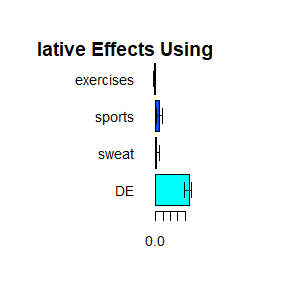The $$summary$$ function returns inference results for all four methods. By default, the relative effects are shown using method 3. To show the results of other methods, we can change by setting the argument $$method$$. Method 4 is calculated for binary/categorical exposures only. If the user would like to see the effect estimations rather than the relative effects, one should set $$RE=F$$.

### Categorical predictor

The following example is given for a categorical exposure: race. In the data set, race takes six categories: empty (not reported), other, mixed, Caucasian, Indian, and African. CAUCASIAN’’ is used as the reference group, each other race group is compared with Caucasian in bmi and the relative effects from mediators are reported by the $$summary$$ function.

test.b.c<- bma.bx.cy(pred=weight_behavior[,3], m=weight_behavior[,c(5:11,12:14)],
y=weight_behavior[,1],cova=weight_behavior[,2],mcov=weight_behavior[,c(2,5)],
mclist = list(1,2),n.iter=500,n.burnin = 100)
#> Compiling model graph
#>    Resolving undeclared variables
#>    Allocating nodes
#> Graph information:
#>    Observed stochastic nodes: 10206
#>    Unobserved stochastic nodes: 72
#>    Total graph size: 31416
#>
#> Initializing model
summary(test.ca.c)
#> Error in summary(test.ca.c): object 'test.ca.c' not found

The jags model fitted for the above model is as following:

#the jags models for the outcomes and mediators
model {temp <- 1:nmc
for(i in 1:N){
mu_y[i] <- beta0 + inprod(c, x[i,]) + inprod(beta,M1[i,]) + inprod(eta,cova[i,])
y[i] ~ dnorm(mu_y[i],prec4) #the final model since y is continuous. The model is changed for
#different format of the outcome, as is shown in the following
#sections
for (j in 1:p1){            #the model for p1 contiuous mediators
mu_M1[i,contm[j]] <- inprod(alpha0.a[j,mind[contm[j],]],mcov[i,temp[mind[contm[j],]]])+inprod(alpha1.a[j,1:c1],x1[i,])
M2[i,contm[j]] ~ dnorm(mu_M1[i,contm[j]],prec1[j])
for (k in contm1[j,1]:contm1[j,2]){
mu_M1_c[i,k] <- inprod(alpha0[k,mind[contm[j],]],mcov[i,mind[contm[j],]])+inprod(alpha1[k,1:c1],x1[i,])
M1[i,k] ~ dnorm(mu_M1_c[i,k],prec2[k])
}
}

for (k in 1:p2){           #the model for p2 binary mediators
logit(mu_M1[i,binm[k]]) <- inprod(alpha0.b[k,mind[binm[k],]],mcov[i,mind[binm[k],]])+inprod(alpha1.b[k,1:c1],x1[i,])
M2[i,binm[k]] ~ dbern(mu_M1[i,binm[k]])
}

for (j in 1:p3){           #the model for p3 categorical mediators
mu_Mc[i,j,1] <- 1 #baseline is the 1st category
for (k in 2:cat2[j]){
mu_Mc[i,j,k] <- exp(inprod(alpha0.c[j,k-1,mind[catm[j],]],mcov[i,mind[catm[j],]])+inprod(alpha1.c[j,k-1,1:c1],x1[i,]))
}
sum_Mc[i,j] <- sum(mu_Mc[i,j,1:cat2[j]])
for (l in 1:cat2[j])
{mu_Mc0[i,j,l] <- mu_Mc[i,j,l]/sum_Mc[i,j]}
M2[i,catm[j]] ~ dcat(mu_Mc0[i,j,1:cat2[j]])
}
}

beta[1:P] ~ dmnorm(mu[1:P], Omega[1:P, 1:P])  #prior distributions for coefficients of model for y
beta0 ~ dnorm(0, 1.0E-6)
c[1:c2] ~ dmnorm(muc[1:c2], Omegac[1:c2,1:c2])
eta[1:cv1] ~ dmnorm(mucv[1:cv1], Omegacv[1:cv1,1:cv1])

for(j in 1:P)     #prior distributions for coefficients of model for not transformed mediators
{alpha1[j,1:c1] ~ dmnorm(mu1.1[j,1:c1], Omega1.1[1:c1, 1:c1])
alpha0[j,1:nmc] ~ dmnorm(mu0.1[j,1:nmc], Omega0.1[1:nmc, 1:nmc])}
for (i in 1:P){
var2[i] ~ dgamma(1,0.1)
prec2[i] <- 1/var2[i]
}

for(j in 1:p1)   #prior distributions for coefficients of model for p1 continuous mediators
{alpha1.a[j,1:c1] ~ dmnorm(mu1.a[j,1:c1], Omega1.a[1:c1, 1:c1])
alpha0.a[j,1:nmc] ~ dmnorm(mu0.a[j,1:nmc], Omega0.a[1:nmc, 1:nmc])}
for (i in 1:p1){
var1[i] ~ dgamma(1,0.1)
prec1[i] <- 1/var1[i]
}

for(j in 1:p2)  #prior distributions for coefficients of model for p2 binary mediators
{alpha1.b[j,1:c1] ~ dmnorm(mu1.b[j,1:c1], Omega1.b[1:c1, 1:c1])
alpha0.b[j,1:nmc] ~ dmnorm(mu0.b[j,1:nmc], Omega0.b[1:nmc, 1:nmc])
}

for (i in 1:p3){  #prior distributions for coefficients of model for p3 categorical mediators
for(j in 1:cat1)
{alpha1.c[i,j,1:c1] ~ dmnorm(mu1.c[j,1:c1], Omega1.c[1:c1, 1:c1])
alpha0.c[i,j,1:nmc] ~ dmnorm(mu0.c[j,1:nmc], Omega0.c[1:nmc, 1:nmc])}
}

var4 ~ dgamma(1,0.1) #the prior for the variance of y when it is continuous
prec4 <-1/var4
}

The rjags model can be revised when different priors or models are to be used. To do that, write the model file and input it to the argument $$filename$$.

### use transfered continuous predictors for y

We can use transformed predictors for the outcome. The following is an example

test.c.c.2<- bma.bx.cy(pred=weight_behavior[,2], m=weight_behavior[,12:14],
y=weight_behavior[,1],fpy=list(1,c("x","x^2")),n.iter=5,n.burnin = 1)
#> Compiling model graph
#>    Resolving undeclared variables
#>    Allocating nodes
#> Graph information:
#>    Observed stochastic nodes: 3894
#>    Unobserved stochastic nodes: 21
#>    Total graph size: 9876
#>
#> Initializing model
summary(test.c.c.2,method=1)
#>
#>  For Predictor 1 :
#> Estimated Relative Effects for Method 1:
#>             DE  sports exercises   sweat
#> mean    1.4115 -0.1352   -0.2591 -0.0172
#> sd      1.3155  0.5665    0.7322  0.0658
#> q_0.025 0.5558 -0.8765   -1.2495 -0.0748
#> q_0.25  0.7419 -0.2557   -0.3562 -0.0737
#> q_0.5   0.8710  0.0165    0.0707 -0.0189
#> q_0.75  1.5406  0.1370    0.1678  0.0376
#> q_0.975 3.1863  0.3480    0.1707  0.0433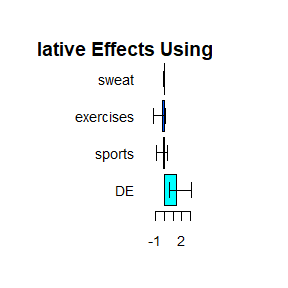We can also have multiple predictors

test.m.c<- bma.bx.cy(pred=weight_behavior[,2:4], m=weight_behavior[,12:14],
y=weight_behavior[,1],n.iter=10,n.burnin = 1)
#> Compiling model graph
#>    Resolving undeclared variables
#>    Allocating nodes
#> Graph information:
#>    Observed stochastic nodes: 3894
#>    Unobserved stochastic nodes: 21
#>    Total graph size: 19194
#>
#> Initializing model
summary(test.m.c,method=3)
#>
#>  For Predictor 1 :
#> Estimated Relative Effects for Method 3:
#>               DE  sports exercises   sweat
#> mean     -0.6613 -0.0017   -0.1010  0.1751
#> sd       26.6446  0.0609    0.4603  0.3235
#> q_0.025 -46.7190 -0.1143   -0.9882 -0.3123
#> q_0.25   -6.7158 -0.0297   -0.1809  0.0993
#> q_0.5     2.3877  0.0172   -0.0711  0.1765
#> q_0.75    3.3672  0.0238    0.1337  0.3256
#> q_0.975  40.3321  0.0742    0.3778  0.7012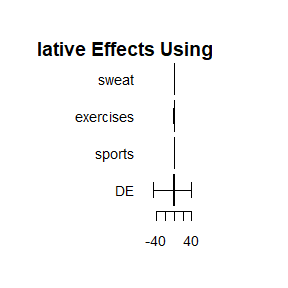#>
#>  For Predictor 2 :
#> Estimated Relative Effects for Method 3:
#>              DE sports exercises   sweat
#> mean    -0.9434 0.1268   -0.0382  0.0300
#> sd       0.7510 0.0716    0.0362  0.0405
#> q_0.025 -2.3663 0.0405   -0.1046 -0.0452
#> q_0.25  -1.1864 0.0763   -0.0557  0.0143
#> q_0.5   -0.7875 0.1015   -0.0281  0.0414
#> q_0.75  -0.5389 0.1584   -0.0105  0.0531
#> q_0.975 -0.0951 0.2424    0.0003  0.0763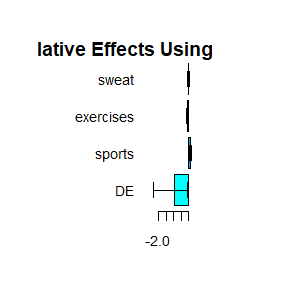#>
#>  For Predictor 3 :
#> Estimated Relative Effects for Method 3:
#>              DE  sports exercises   sweat
#> mean     0.2679 -0.0387    0.0014 -0.0103
#> sd       1.1085  0.1655    0.0094  0.0193
#> q_0.025 -1.6786 -0.1743   -0.0138 -0.0435
#> q_0.25   0.0478 -0.1583   -0.0007 -0.0202
#> q_0.5    0.4072 -0.0730    0.0029 -0.0091
#> q_0.75   0.9715 -0.0589    0.0061  0.0050
#> q_0.975  1.7554  0.2714    0.0143  0.0119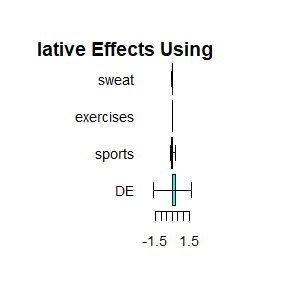#>
#>  For Predictor 4 :
#> Estimated Relative Effects for Method 3:
#>             DE  sports exercises   sweat
#> mean    0.1487 -0.0042    -7e-04 -0.0399
#> sd      0.1329  0.0019     7e-04  0.0289
#> q_0.025 0.0142 -0.0067    -2e-03 -0.0701
#> q_0.25  0.0771 -0.0055    -9e-04 -0.0548
#> q_0.5   0.1346 -0.0041    -7e-04 -0.0430
#> q_0.75  0.1584 -0.0034    -2e-04 -0.0374
#> q_0.975 0.4126 -0.0012     0e+00  0.0172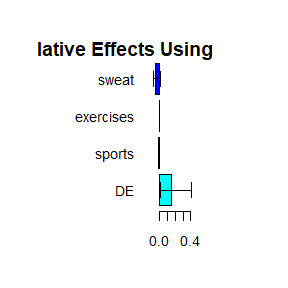#>
#>  For Predictor 5 :
#> Estimated Relative Effects for Method 3:
#>                 DE    sports exercises   sweat
#> mean     -241.9611  -40.7378    1.4841  4.7982
#> sd        712.7054  119.2273    3.5949 14.9380
#> q_0.025 -1718.6179 -288.0206   -0.0742 -0.9395
#> q_0.25     -8.7775   -1.5061   -0.0009 -0.1116
#> q_0.5      -0.3918   -0.1493    0.0479 -0.0206
#> q_0.75     -0.0334   -0.0153    0.4626  0.0082
#> q_0.975     0.6392    0.2791    9.0949 35.7088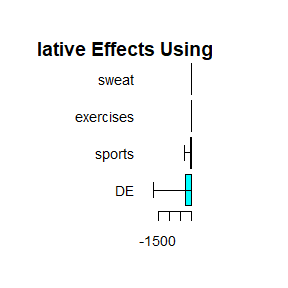#>
#>  For Predictor 6 :
#> Estimated Relative Effects for Method 3:
#>              DE  sports exercises   sweat
#> mean    -0.2879 -0.0625    0.0291 -0.0535
#> sd       2.7421  0.3512    0.1473  0.1783
#> q_0.025 -6.0626 -0.7946   -0.0819 -0.4258
#> q_0.25  -0.0200 -0.0488   -0.0590 -0.0366
#> q_0.5    0.7389  0.1014   -0.0044  0.0210
#> q_0.75   1.0562  0.1265    0.0063  0.0462
#> q_0.975  1.4355  0.1674    0.3351  0.0653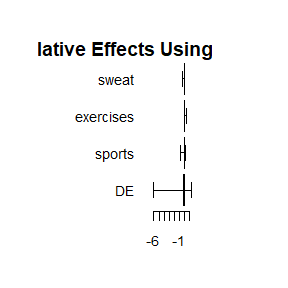#>
#>  For Predictor 7 :
#> Estimated Relative Effects for Method 3:
#>             DE sports exercises   sweat
#> mean    0.8561 0.0880   -0.0528  0.1087
#> sd      0.2282 0.0866    0.0378  0.1618
#> q_0.025 0.3791 0.0205   -0.0957 -0.0212
#> q_0.25  0.8664 0.0436   -0.0863  0.0204
#> q_0.5   0.9287 0.0552   -0.0518  0.0678
#> q_0.75  0.9657 0.0998   -0.0285  0.1204
#> q_0.975 0.9918 0.2655    0.0019  0.4418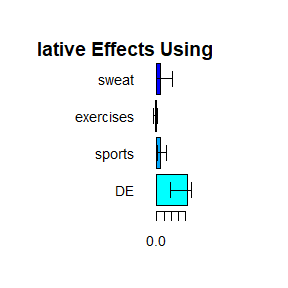### Binary outcome

The following is an example for binary outcome overweight (yes or no)

test.m.b<- bma.bx.cy(pred=weight_behavior[,3], m=weight_behavior[,12:14],
y=weight_behavior[,15],cova=weight_behavior[,5],n.iter=500,n.burnin = 100)
#> Compiling model graph
#>    Resolving undeclared variables
#>    Allocating nodes
#> Graph information:
#>    Observed stochastic nodes: 3930
#>    Unobserved stochastic nodes: 22
#>    Total graph size: 8973
#>
#> Initializing model
summary(test.m.b,method=2)
#>
#>  For Predictor 1 :
#> Estimated Relative Effects for Method 2:
#>              DE sports exercises   sweat
#> mean     0.6966 0.2753   -0.0225  0.0506
#> sd       0.9125 0.8780    0.0900  0.1412
#> q_0.025 -0.6383 0.0398   -0.1533 -0.0342
#> q_0.25   0.6858 0.1293   -0.0359  0.0022
#> q_0.5    0.8002 0.1897   -0.0118  0.0247
#> q_0.75   0.8644 0.2878    0.0008  0.0545
#> q_0.975  0.9624 1.1567    0.0383  0.3336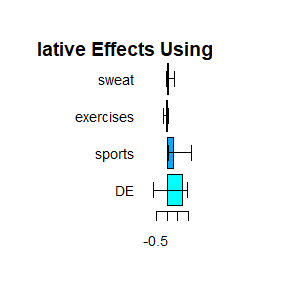For such case, the model for y in the jags is set as following by default, which can be revised.

    logit(mu_y[i]) <- beta0 + inprod(c, x[i,]) + inprod(beta,M1[i,]) + inprod(eta,cova[i,])
y[i] ~ dbern(mu_y[i])

### Time-to-Event outcome

The following is an example for survival model

test.m.t.1<- bma.bx.cy(pred=example2[,"x"], m=example2[,"M"],  y=Surv(example2[,"time"],example2[,"status"]), inits=function(){  list(r=1,lambda=0.01)},n.iter=10,n.burnin = 1)
#> Compiling model graph
#>    Resolving undeclared variables
#>    Allocating nodes
#> Graph information:
#>    Observed stochastic nodes: 300
#>    Unobserved stochastic nodes: 12
#>    Total graph size: 2861
#>
#> Initializing model
temp1=summary(test.m.t.1)
print(temp1,method=1,RE=FALSE)
#>
#>  For Predictor 1 :
#> Estimated Effects for Method 1:
#>              TE     DE      m1
#> mean     0.5985 0.0795  0.5190
#> sd       0.5424 0.0321  0.5234
#> q_0.025 -0.5014 0.0462 -0.5476
#> q_0.25   0.5278 0.0462  0.4815
#> q_0.5    0.7611 0.1025  0.6587
#> q_0.75   0.8470 0.1025  0.8008
#> q_0.975  1.0878 0.1171  0.9707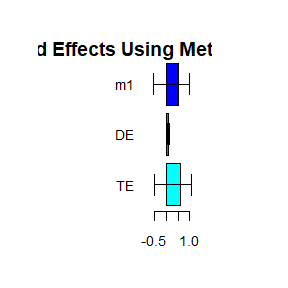For such case, the model for y in the jags is set as following by default, which can be revised.

elinpred[i] <- exp(inprod(c, x[i,]) + inprod(beta,M1[i,]))
base[i] <- lambda*r*pow(y[i,1], r-1)
loghaz[i] <- log(base[i]*elinpred[i])
phi[i] <- 100000-y[i,2]*loghaz[i]-log(exp(-lambda*pow(y[i,1],r)*elinpred[i])-exp(-lambda*pow(tmax,r)*elinpred[i])) +log(1-exp(-lambda*pow(tmax,r)*elinpred[i]))
zero[i]  ~ dpois(phi[i])

The following is an example of setting the priors for r and lambda:

r ~ dunif(0,10) #  dunif(1,1.2)
lambda ~ dgamma(1,0.01)

### Categorical outcome

Finally, the following is an example for categorical outcomes

test.m.c<- bma.bx.cy(pred=weight_behavior[,2:4], m=weight_behavior[,12:13],
y=as.factor(weight_behavior[,14]),cova=weight_behavior[,5],n.iter=5,n.burnin = 1)
#> Compiling model graph
#>    Resolving undeclared variables
#>    Allocating nodes
#> Graph information:
#>    Observed stochastic nodes: 2592
#>    Unobserved stochastic nodes: 20
#>    Total graph size: 23800
#>
#> Initializing model
summary(test.m.c,method=3)
#>
#>  For Predictor 1 outcome 1 :
#> Estimated Relative Effects for Method 3:
#>          DE sports exercises
#> mean    NaN    NaN       NaN
#> sd       NA     NA        NA
#> q_0.025  NA     NA        NA
#> q_0.25   NA     NA        NA
#> q_0.5    NA     NA        NA
#> q_0.75   NA     NA        NA
#> q_0.975  NA     NA        NA
#> Error in plot.window(xlim, ylim, log = log, ...): need finite 'xlim' valuesFor such case, the model for y in the jags is set as following by default, which can be revised.

mu_y1[i,1] <- 1
for (k in 2:caty)  #caty is the number of categories of y
{mu_y1[i,k] <- exp(beta0[k-1] + inprod(c[k-1,], x[i,]) + inprod(beta[k-1,],M1[i,]) + inprod(eta[k-1,],cova[i,]))}
sum_y[i] <- sum(mu_y1[i,1:caty])
for (l in 1:caty)
{mu_y[i,l] <- mu_y1[i,l]/sum_y[i]}
y[i] ~ dcat(mu_y[i,])